# 3 Equations

## Commands

Latex allows you to quickly write mathematical equations by specifying characters using commands you can type using the keyboard. For example, we can write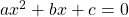using the code

[‌latex] ax^2 + bx + c = 0 [/latex]

While some common math symbols like +/- and exponentiation have a single symbol on the keyboard, most commands start with “\” and then describe the command. An example of an equation that requires \ commands is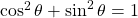, which requires commands for the sine, cosine, and angle:

[‌latex] \cos^2 \theta + \sin^2 \theta = 1 [/latex]

The table below shows some common “\” commands for symbols, in addition to the trigonometric functions and Greek letters used in the previous example.

 Algebra Calculus Logic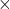\times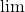\lim\neg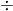\div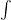\int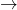\rightarrow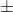\pm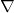\nabla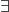\exists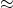\approx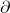\partial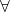\forall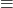\equiv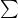\sum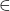\in

Commands can also take arguments using curly brackets. For example, the fraction and square root in the quadratic formula both take arguments.

[‌latex] x = \frac{-b \pm \sqrt{b^2 + 4ac}}{2a} [/latex]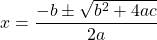Superscripts and subscripts are also considered arguments. Use ^{} to create a superscript. If, no brackets are used, only the first character will be part of the superscript.
[‌latex]e^{i \pi} = -1[/latex]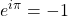Use _{} to create a subscript. Again, if no brackets are used only the first character will be part of the subscript.

[‌latex] x_1 + x_2 = 2x_3[/latex]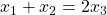You can add a subscript and a superscript to the same item. The order of the subscript and superscript does not matter. For example:

[‌latex]\int_{a}^{b} u dv = u(x)v(x)|_a^b - \int^{b}_a v du [/latex]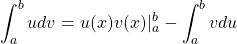Latex is generally not sensitive to white space when typesetting math. Outside of Pressbooks, the commands \;, \:, \, explicitly add large, medium, and small spaces respectively. However, these commands do not seem to be compatible with the QuickLaTeX plugin. As a workaround, you can use the command \hspace{} to add a specific amount of horizontal white space. For example, we can add a space between the functions and the differential variable:

[‌latex]\int_{a}^{b} u \hspace{2pt} dv = u(x)v(x)|_a^b \int^{b}_a v \hspace{2pt} du[/latex]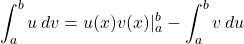### Errors

If a statement is not entered correctly, the equation may not display as expected or QuickLaTeX may display an error. Errors take practice to read. For example, if I were to try to write the quadratic formula as

[‌latex] x = \frac{-b \pm \sqrt{b^2 + 4ac}{2a} [/latex]

I would get the error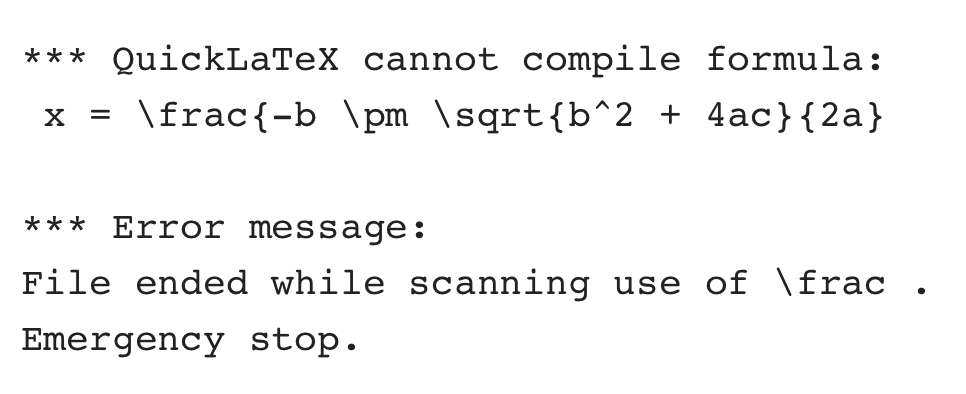Try to spot what is causing the error. The answer is given in the solutions to the exercises for this section.